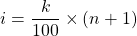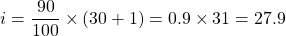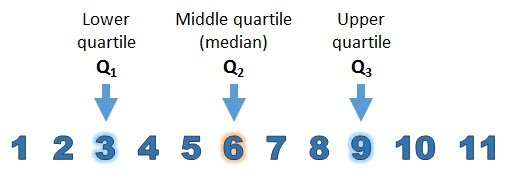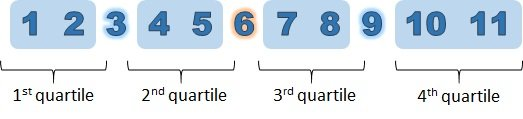# Medians, Percentiles, Quartiles & Interquartile Range

in STEMGeeks8 days ago

Hi there and welcome. In this statistics post I cover medians, percentiles, quantiles and the interquartile range. These topics are introductory and simple but they can be informative in understanding numerical data.Pixabay Image Source

## Topics

• Median As The Middle Number
• What Is A Percentile?
• Quartiles
• Interquartile Range As The Middle 50% Of Values

## Median As The Middle Number

Given a sorted list of numbers from data, the median number is the middle number. Half of the numbers are below this middle number and the other half of numbers are above this middle number.

Example One

From the number list of 8, 10, 2, 3, 7, 9 and 11, what is the median?

Sorting the numbers gives `2, 3, 7, 8, 9, 10, 11`. The median/middle number here is 8.

Example Two

What if we have an even amount of numbers? How would the median be determined? With an even amount of numbers, take the middle two numbers and find the average of the two.

In the list 38, 22, 21, 19, 84, 75, 34 and 41 the sorted list would be `19, 21, 22, 34, 38, 41, 75, 84`. There are eight numbers in this sorted list so the two middle numbers have to be taken. The two middle numbers in the sorted list are 34 and 38. Taking the average of 34 and 38 gives 36. The median number here is 36 which does not appear in the original list (and that is okay).Pixabay Image Source

## What Is A Percentile?

A percentile is a measure which represents a value where the percentage of data (observations) that falls below it. As an example, the 80-th percentile represents a value where 80% of the observed (sorted) values fall below it.

A test score of 90 is very good in a lot of cases. A test score of 72 can be considered very good if that score of 72 is the 95-th percentile of grade scores for a test. The 72 score is better than 95% of the scores below it.

Computing A Percentile

Source/Reference: Introductory Statistics OpenStax ©2018 Rice University Page 92-93

There is a way to find a value in the k-th percentile from a sorted (ascending) list of values. Let `k` be k-th percentile, (Having k = 80 represents the 80th percentile), let `i` be the index or position of a value and let `n` be the number of values in the sorted list.

Given a percentile `k`, the index (position) formula is given by:(Rendered with QuickLaTeX.com)

If `i` is an integer (no decimals) then the value for the `k-th` percentile is located in the position `i` of the sorted list.

With the case of `i` not being an integer, consider the next integer below `i` and the next integer above `i`. Use these two `i` indices to obtain values in their respective positions. The k-th percentile is given by the average of these two values.

Example Of Computing A Percentile

Consider a list of 30 test scores.

`74 77 67 83 73 61 64 71 58 51 80 52 60 84 81 70 79 53 50 69 65 86 88 76 54 57 75 87 66 82`

Sorting the above list gives this:

`50 51 52 53 54 57 58 60 61 64 65 66 67 69 70 71 73 74 75 76 77 79 80 81 82 83 84 86 87 88`

What is the 90th percentile here?

We have `n = 30`, `k = 90`. Use these in the index `i` formula.This `i` value is 27.9, a non-integer. The next integer below 27.9 (rounded down) is 27 and the next integer above 27.9 is 28. Locate the 27th number and the 28th number in the sorted list.

The 27th number is 84 and the 28th number is 86. Taking the average of these two numbers is 85. The 90th percentile of the test scores is 85. Ninety percent of values (27 values) are below 85.Pixabay Image Source

## Quartiles

The word quartile contains the word quart which is also contained in the word quarter. Quartiles split the list of sorted values into quarters. The first quartile refers to the first twenty-five percent of values, the second quartile is for the next 25 percent and so on until the fourth quartile (last 25% of values).Website & Image Source

The image above refered to quartiles as cutoff points while this next image refers to quartiles as quarters.Website & Image Source

## Interquartile Range As The Middle 50% Of Values

Knowing the concepts of percentiles and quartiles leads up to this concept of the interquartile range (IQR for short). The IQR is the difference between the upper quartile Q3 cutoff value and the lower quartile Q1 cutoff value.

`IQR = Q3 - Q1`

This interquartile range is the range of values of the middle fifty percent of the sorted list of values. Another way to say this is that the IQR is the difference of the highest value and the lowest value in the middle 50% of values.

Example

Let's revisit the sorted list from the percentiles example.

`50 51 52 53 54 57 58 60 61 64 65 66 67 69 70 71 73 74 75 76 77 79 80 81 82 83 84 86 87 88`

The 75-th percentile (Q3) is 79.75 and the 25th percentile (Q1) is 60.25. Taking the difference of these two values gives the IQR of 19.5.
(I used the statistical programming language R to do the calculations. Doing the hand calculations takes a bit of time.)Pixabay Image Source